# 摘要

【关键词】：同态加密，多方安全计算，联邦学习，隐私计算

# 1 背景知识

## 1.3 中国剩余定理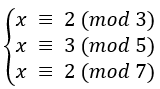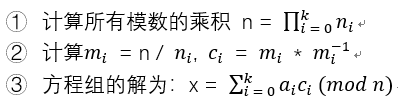# 2 Paillier算法原理

## 2.1 Paillier简介

• 以RSA为代表的，基于大数因数分解难题的公钥加密算法
• 以ElGama为代表的，基于大数离散对数难题的公钥加密算法

Paillier加密算法，由Pascal Paillier于1999年发表，给出了公钥加密算法的一个新的分支领域。Paillier基于复合剩余类难题，满足加法同态和数乘同态，具有非常高效的运行时性能。

## 2.2 一个典型的应用场景## 2.3 Paillier算法

### 2.3.1 密钥生成p = 11 q = 19 n = 209 λ = 90 g = 147 μ = 153

Alice将 n 和g 封装成公钥 public-key = (n, g)

### 2.3.2 加密

• Bob选择一个随机数r,满足0 < r < n
• Bob计算加密后的密文 c = gm.rn mod n2
m = 8 r = 3 n_square = pow(n, 2) # n_square = 43681 c = gmpy2.mod(pow(g, m)*pow(r, n), n_square) # c = 32948

### 2.3.3 解密

Alice计算明文

m = L(cλ mod n2) * μ mod n

c = 32948 m = gmpy2.mod(L(gmpy2.mod(pow(c, lam), n_square), n) * mu, n) # m = 8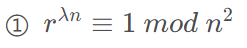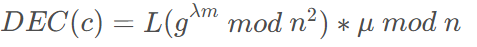② gλ mod n2 = (1 + naλ) * bnλ mod n2 = 1 + aλn mod n2

DEC(c) = L( (1 + naλ) * bnλ mod n2 ) * μ mod n = L(1 + amλ*n mod n2) * μ mod n

μ = L(gλ mod n2)-1 mod n = L(1 + aλn mod n2)-1 mod n

DEC(c) = (amλ mod n2) * (a * λ mod n2)-1 mod n = m

### 2.3.4 同态加

c = c1 * c2 mod n2

Alice使用私钥计算DEC(c) = m1 + m2

c = ((gm1rn mod n2) * (gm2rn mod n2)) mod n2 = (gm1rngm2rn) mod n2 = (gm1 + m2r2n) mod n2 = ENC(m1 + m2)

DEC(c) = DEC(ENC(m1 + m2)) = m1 + m2

c1 + m2 = (gm1rn mod n2 * gm2 mod n2) mod n2 = gm1+krn mod n2 = E(m1+m2)

### 2.3.5 同态数乘

Paillier算法目前只支持明文和密文相乘的计算方式，不支持密文和密文相乘。

Bob使用Alice对明文m1加密后的密文c1和明文k，计算

c = c1k mod n2

Alice使用私钥计算DEC(c) = k*m1

c = c1k mod n2 = (gm1*rn mod n2)k mod n2 = gk*m1* r1n mod n2 = E(k*m1)

DEC(c) = DEC(ENC(k * m1)) = k * m1

## 2.4 算法优化

### 2.4.1 参数g优化

μ = L(gλ mod n2)-1 mod n = L((n + 1)}λ mod n2)}-1 mod n = L((1 + λ * n) mod n2)-1 mod n = λ-1 mod n

μ 生成可以直接取λ 对 n的模反元素。

c = (n*m+1)*rn mod n2

### 2.4.2 高阶幂运算优化

λ = (p - 1)(q - 1) / 2

hs = hns mod ns + 1

c = (n * m + 1)hsα mod ns + 1

### 2.4.3 使用中国剩余定理

1. 定义分解因子p和q对应的函数 Lp(x) = (x-1) / p, Lq(x) = (x - 1) / q
2. 计算hp ≡ (p - 1) * q (mod p), hq ≡ (q - 1) * p (mod q)
3. 计算mp = Lp(cp - 1 mod p2) hp mod p, mq = Lq(cq - 1 mod q2) hq mod q
4. 计算m = CRT(m_p, m_q) mod n, m 即为使用中国剩余定理优化的解密后的明文

### 2.4.4 性能优化对比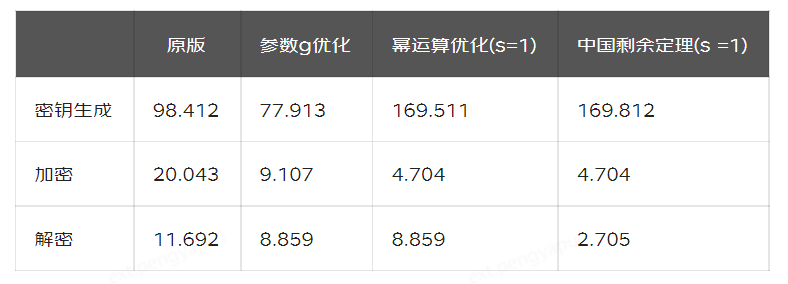# 3 功能扩展

## 3.1 浮点数支持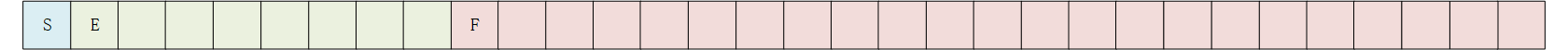IEEE 754标准中，单精度浮点表示采用1位符号，8位阶码和23位分数。

x = -1sign * (1 + Fraction) * 2(Exponent - Bias)

## 3.2 负数支持

• 当 m1 + m2 >= 0时，DEC(c) = m1 + m2
• 当-n <= m1 + m2 < 0时， DEC(c) = m1 + m2 + n
• 当m1 + m2 < -n 时，计算结果不正确

• 当k * m1 >= 0 , 时DEC(c) = k * m1
• 当-n <= k * m1 < 0时，DEC(c) = k * m1 + n
• 当k * m1 < -n时，计算结果不正确

1. 操作数在参与同态计算前对n取模，用来统一正数可以直接参与计算，负数则需要补n再进行计算的问题。
2. 设定操作数的最大值MAX_VALUE，比如32位整型的最大值。并使得n的取值大于3 * MAX_VALUE，这样使得对于合法的m1和m2，不存在m1+ m2 < -n的场景，且m1 + m1 < 0时，计算结果总是大于MAX_VALUE。

# 4 主要公钥加密算法对比

Paillier，RSA和ELGamal算法均为典型的基于公钥的加密算法，我们从功能指标和性能指标两个方向，来对比这些加密算法的区别和联系。

## 4.1 功能指标对比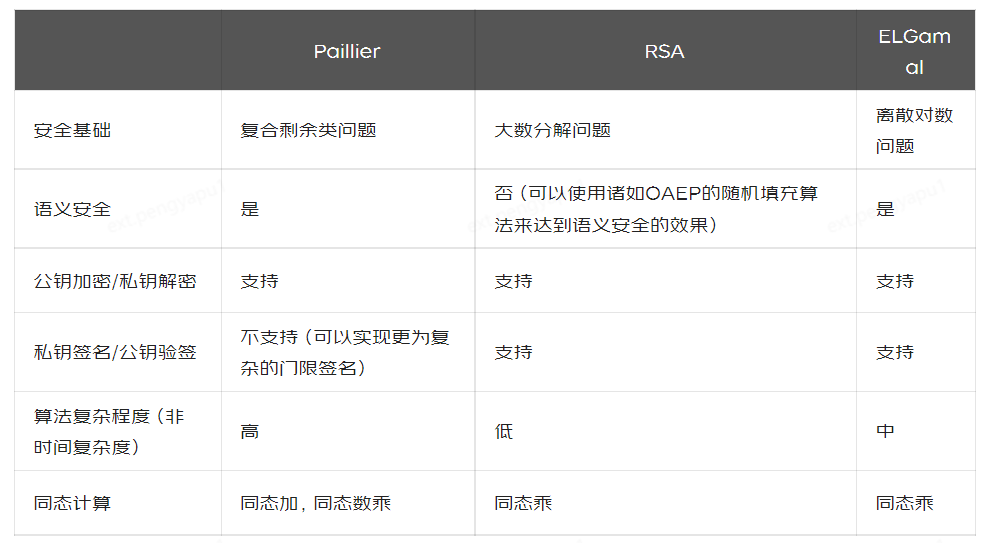## 4.2 性能指标对比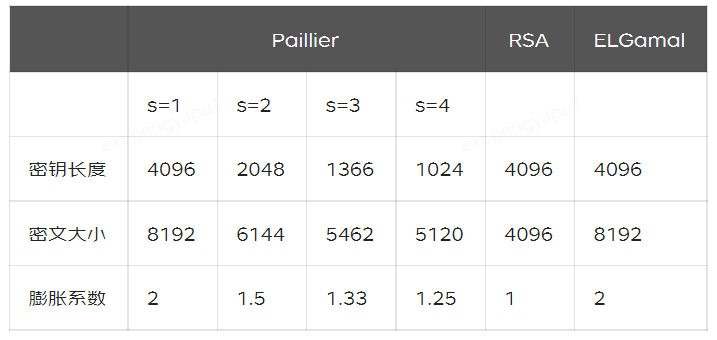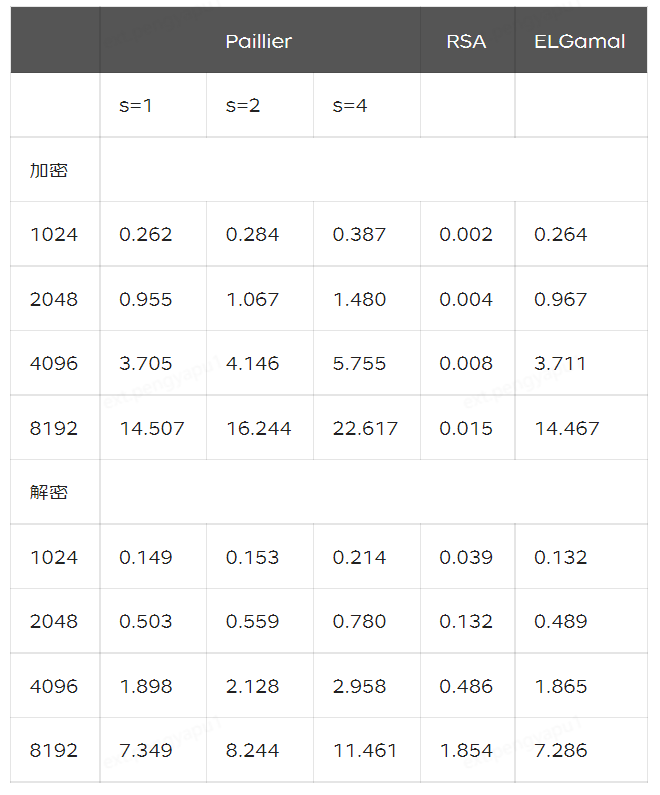# 5 同态加密的应用# 参考文献

 P. Paillier, “Public-key cryptosystems based on composite degree residuosity classes,” in Proceedings of Advances in Cryptology (EUROCRYPT’99),pp. 223–238, Prague, Czech Republic, May 1999.

 Zhu, L., Liu, Z., Han, S.: Deep leakage from gradients. In: Annual Conference on Neural Information Processing Systems (NeurIPS) (2019)

 D. Choi, S. Choi, and D. Won, “Paillier’s cryptosystem revisited,” in Proceedings of the 8th ACM Conference on Computer and Communications Security(CCS’01), pp. 206–214, Philadelphia, Pennsylvania, USA, Nov. 2001

 I. Damgard and M. Jurik. A generalization, of Paillier"s Eurocrypt "99, volume 1592 of LNCS. IACR,Springer-Verlag, 1999.

 I. Damg˚ard, J. Jurik, and J. Nielsen, “A generalization of paillier’s public-key system with applications to electronic voting,” International Journal of Information Security, no. 9, pp. 371–385, 2010.

 Cao, Z. and Liu, L., "The Paillier"s Cryptosystem and Some Variants Revisited." arXiv preprint arXiv:1511.05787,2015.